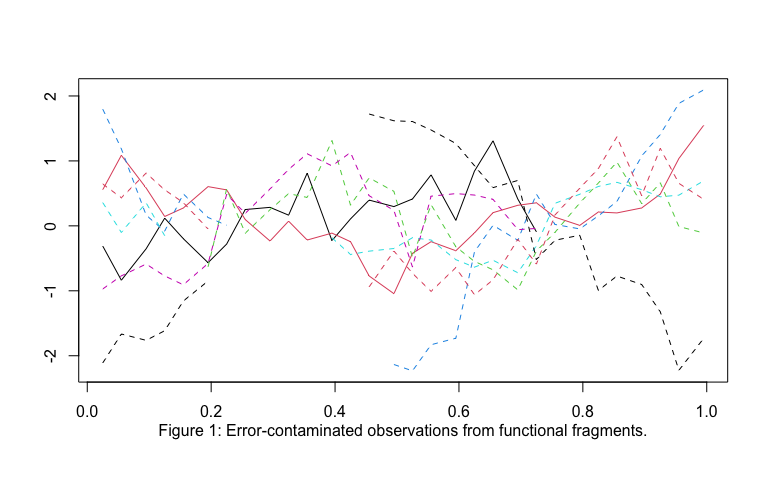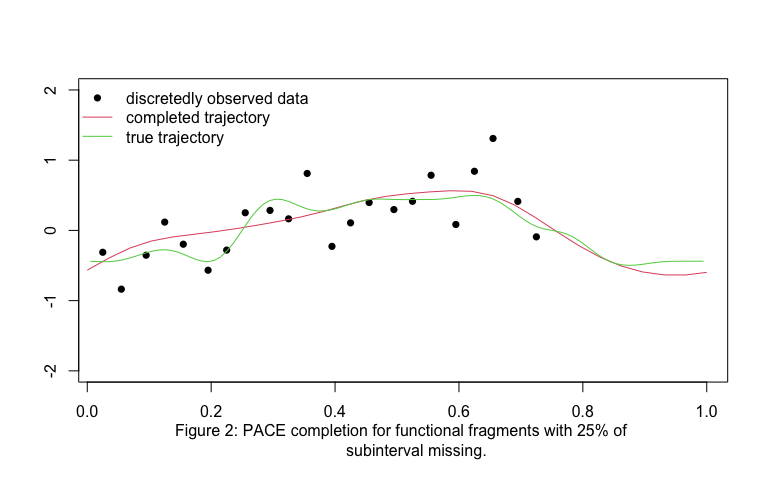# Completion of Functional Fragments

## Overview

We utilize the package fdapace (Gajardo et al. 2021) to showcase the completion of simulated functional fragments when one has available a densely observed sample of curves (Carroll et al. 2020).

### Simulate functional fragments

set.seed(1)
# set parameters for simulation
n <- 50 # number of sample trajectories
m <- 30 # number of observation per curve
grid <- seq(0, 1, len = m) # set up regular grid
lambda.cos <- 3^(-(2*(1:25)-1)) # eigenvalues
lambda.sin <- 3^(-(2*(1:25)))
mpct <- 0.25 # proportion of domain missing
densegr <- ((1:100) - .5)/100 # dense regular grid to generate the data
gr <- densegr[(100/m)*(1:m)] # observation grid
sigma2 <- 0.1 # variance for noise term

# generate discretely observed complete functional data
x.full <- matrix(0, n, length(densegr))
for (j in 1:length(lambda.cos)) {
f <- sqrt(lambda.cos[j])*sqrt(2)*cos(2*pi*(2*j - 1)*densegr)
x.full <- x.full + rnorm(n, 0, 1)%*%t(f)
}
for (j in 1:length(lambda.sin)) {
f <- sqrt(lambda.sin[j])*sqrt(2)*sin(2*pi*(2*j)*densegr)
x.full <- x.full + rnorm(n, 0, 1)%*%t(f)
}

# subset the original dense true curve to the k-equidistance sampling scheme;
x <- x.full[, densegr %in% gr]

# generate observation periods by deleting a random subinterval from domain,
# with 20% of data is complete
# matrix of indicators for inclusion/exclusion of observation
x.obs <- matrix(1, n, length(grid))
centers <- c(0.25, 0.5, 0.75)
x.obs[1, ] <- grid <= 0.75
for (i in floor(n*0.2):n) {
cen <- runif(1)
x.obs[i, (cen - mpct/2 < grid)&(grid < cen + mpct/2)] <- FALSE
# missing interval = (cen-u,cen+u)
}
# remove missing periods
x[!x.obs] <- NA

# distort by error term
for(i in 1:dim(x)){
for(j in 1:dim(x)){
x[i,j] <- x[i,j] + rnorm(n = 1, sd = sqrt(sigma2))
}
}
# visualize the functional fragments
matplot(gr, t(x[c(1, 9:15), ]), type="l", lty=c(1, 1, 2, 2, 2, 2, 2, 2),
xlab="", ylab="")
title(sub = 'Figure 1: Error-contaminated observations from functional fragments.',
line = 2)### Use FPCA to complete the missing fragments

# convert x matrix into 3 vectors
id <- c()
time <- c()
y <- c()
for(i in 1:n){
id <- append(id, rep(i, sum(x.obs[i, ])))
time <- append(time, grid[x.obs[i, ] == 1])
y <- append(y, x[i, x.obs[i,] == 1])

}
L3 <- MakeFPCAInputs(IDs = id, tVec = time, y)
optns <- list(dataType = 'Sparse', usergrid = TRUE, methodXi = 'CE')
fit <- FPCA(L3$Ly, L3$Lt, optns = optns)
# completed trajectory for the 1st test sample
PACE.pred <- as.vector(predict(fit, newLy = L3$Ly, newLt = L3$Lt,
K = fit$selectK, xiMethod = "CE")$predCurves)
# Visualize and compare the observations, true trajectory and completed trajectory
# scatterplot of original data with missing parts
matplot(gr, x[1, ], pch = c(16), col = 1, xlab = "", ylab = "", ylim = c(-2, 2))
lines(grid, PACE.pred, lty = 1, col = 2, xlab = "", ylab = "")# completed functional data
lines(densegr, x.full[1, ], col = 3)# discretely observed complete functional data
legend("topleft", legend = c("discretedly observed data", "completed trajectory",
"true trajectory"),
bty = "n", lty = c(NA, 1, 1), pch = c(16, NA, NA), col = 1:3)
title(sub = 'Figure 2: PACE completion for functional fragments with 25% of
subinterval missing.', line = 3)